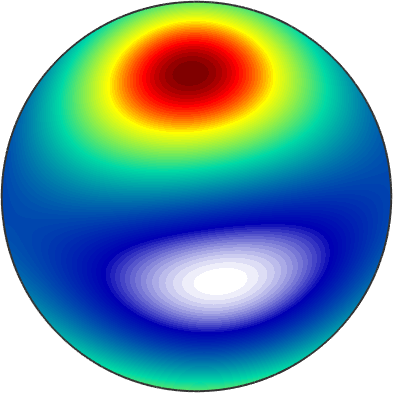Tensor Arithmetics edit page

MTEX offers powerful functionalities to calculate with tensors and lists of tensors without the need of many nested loops.

## Basic algebra

First of all, all basic operations like *, .*, +, -, .^ known from linear algebra work also on lists of tensors.

## Tensor Products

Tensor product are the canonical way how tensors interact with each other. As an example consider a rank 4 stiffness tensor

Then by Hooks law the stiffness tensor acts on a strain tensor, e.g.,

according to the formula

$\sigma_{ij} =\sum_{k,l} C_{ijkl} \epsilon_{kl}$

and turns it into the stress tensor $$\sigma$$. In MTEX such tensor products can be computed in its most general form by the command EinsteinSum.

here the negative numbers indicate the indices which are summed up. Each pair of equal negative numbers corresponds to one sum. The positive numbers indicate the order of the dimensions of the resulting tensor. Accordingly we can compute the outer product

$(a \otimes b)_{ij} = a_i b_j$

beween two rank one tensors

by the command

and the inner product

$a \cdot b = \sum_i a_i b_i$

by

As a final example we consider the linear compressibility in a certain direction v which can be computed by the formula

$c = \sum_{i,j,k} S_{ijkk} v_i v_j$

where $$C = S^{-1}$$ is the inverse of the comcompliance thensor, i.e. the stiffness tensor

Here we used the inv to compute the inverse of any rank 2 or rank 4 tensor. There are shortcuts in MTEX for specific tensor products. E.g. the relation between stress and strain can be more compactly written as a edouble dot product

The double dot product between two rank two tensors is essentially their inner product and can be equivalently computed from the trace of their matrix product

## Determinant

For rank two tensors we can compute the determinant of the tensor by the command det

## Rotating a tensor

Rotation a tensor is done by the command rotate. Let's define a rotation

Then the rotated tensor is given byHere is another example from Nye (Physical Properties of Crystals, p.120-121) for a third-rank tensor